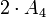# Group cohomology of special linear group:SL(2,3)

View group cohomology of particular groups | View other specific information about special linear group:SL(2,3)

## Family contexts

Family name Parameter values General discussion of group cohomology of family
double cover of alternating group degree$n = 4$, i.e., the group$2 \cdot A_4$ group cohomology of double cover of alternating group
special linear group of degree two over a finite field of size$q$$q = 3$, i.e., field:F3, i.e., the group is$SL(2,3)$ group cohomology of special linear group of degree two over a finite field

## Homology groups for trivial group action

FACTS TO CHECK AGAINST (homology group for trivial group action):
First homology group: first homology group for trivial group action equals tensor product with abelianization
Second homology group: formula for second homology group for trivial group action in terms of Schur multiplier and abelianization|Hopf's formula for Schur multiplier
General: universal coefficients theorem for group homology|homology group for trivial group action commutes with direct product in second coordinate|Kunneth formula for group homology

### Over the integers

The homology groups over the integers are as follows:$H_m(SL(2,3);\mathbb{Z}) = \left \lbrace \begin{array}{rl} \mathbb{Z}, & m = 0 \\ \mathbb{Z}/3\mathbb{Z}, & m \equiv 1 \pmod 4 \\ \mathbb{Z}/24\mathbb{Z}, & m \equiv 3 \pmod 4 \\ 0, & m \mbox{ even}, m > 0 \\\end{array}\right.$

The homology groups have a period of 4, which is in keeping with the fact that$SL(2,3)$ is a finite group with periodic cohomology.

## GAP implementation

### Computation of homology groups

The homology groups for trivial group action on the integers can be computed using the Hap package (if the package is installed but not automatically loaded, load it using LoadPackage("hap");), specifically its GroupHomology function. The function returns a list of numbers which are the orders of cyclic groups whose external direct product is the desired homology group.

#### First homology group

The first homology group, which is also the abelianization, can be computed as follows:

gap> GroupHomology(SL(2,3),1);
[ 3 ]

This says that$H_1(SL(2,3);\mathbb{Z}) = \mathbb{Z}/3\mathbb{Z}$.

#### Second homology group

The second homology group, which is also the Schur multiplier, can be computed as follows:

gap> GroupHomology(SL(2,3),2);
[  ]

This says that$H_2(SL(2,3);\mathbb{Z}) = 0$.

#### First few homology groups

gap> List([1..8],i -> [i,GroupHomology(SL(2,3),i)]);
[ [ 1, [ 3 ] ], [ 2, [  ] ], [ 3, [ 8, 3 ] ], [ 4, [  ] ], [ 5, [ 3 ] ],
[ 6, [  ] ], [ 7, [ 8, 3 ] ], [ 8, [  ] ] ]